# NCERT Exemplar Class 12 Chemistry Solutions for Chapter 8 - The D Block And F Block Elements

NCERT Class 12 Chemistry Exemplar for Chapter 8 D and F Block elements help you to have complete knowledge of the concepts involved in the chapter. Studying the D Block and F Block elements are very much important to score good marks in chemistry examination and to pursue a career in the field of chemistry. To master the concepts of this chapter students are advised to solve the NCERT exemplar Class 12 Chapter 8 D block and F Block elements.

Solving these questions will help your preparation for competitive examination and entrance examinations like JEE, JEE Advanced NEET and state board entrance tests.

## Download PDF of NCERT Exemplar For Class 12 Chemistry Chapter 8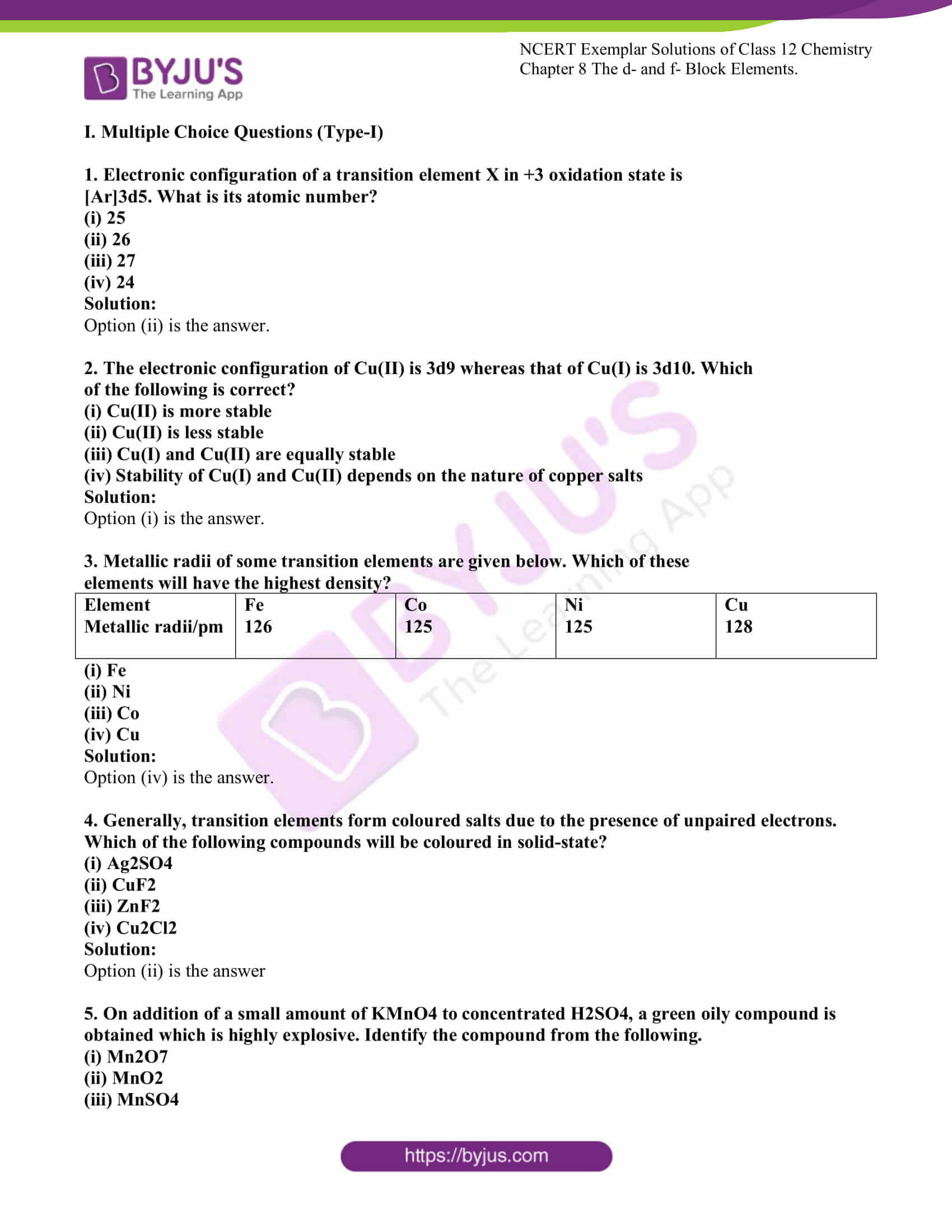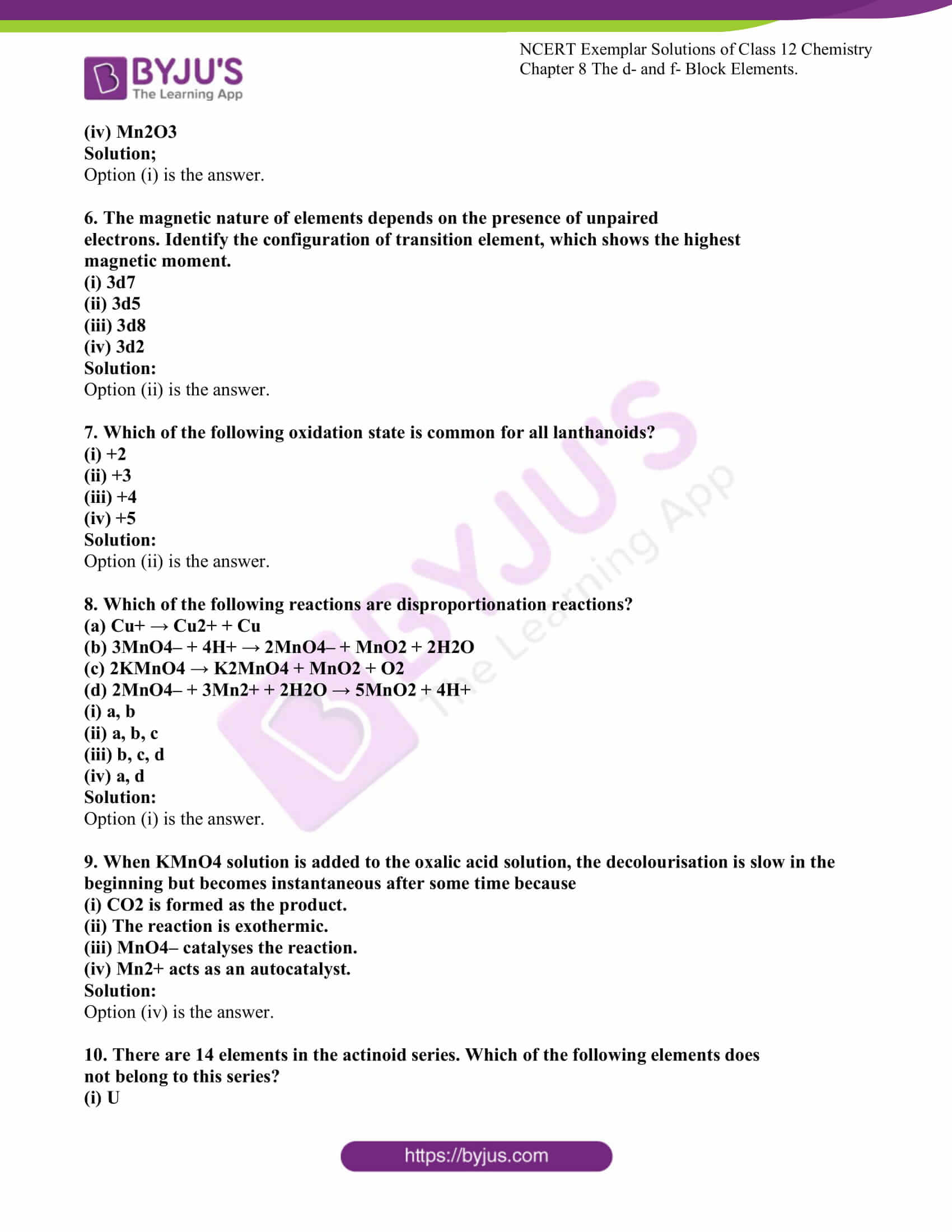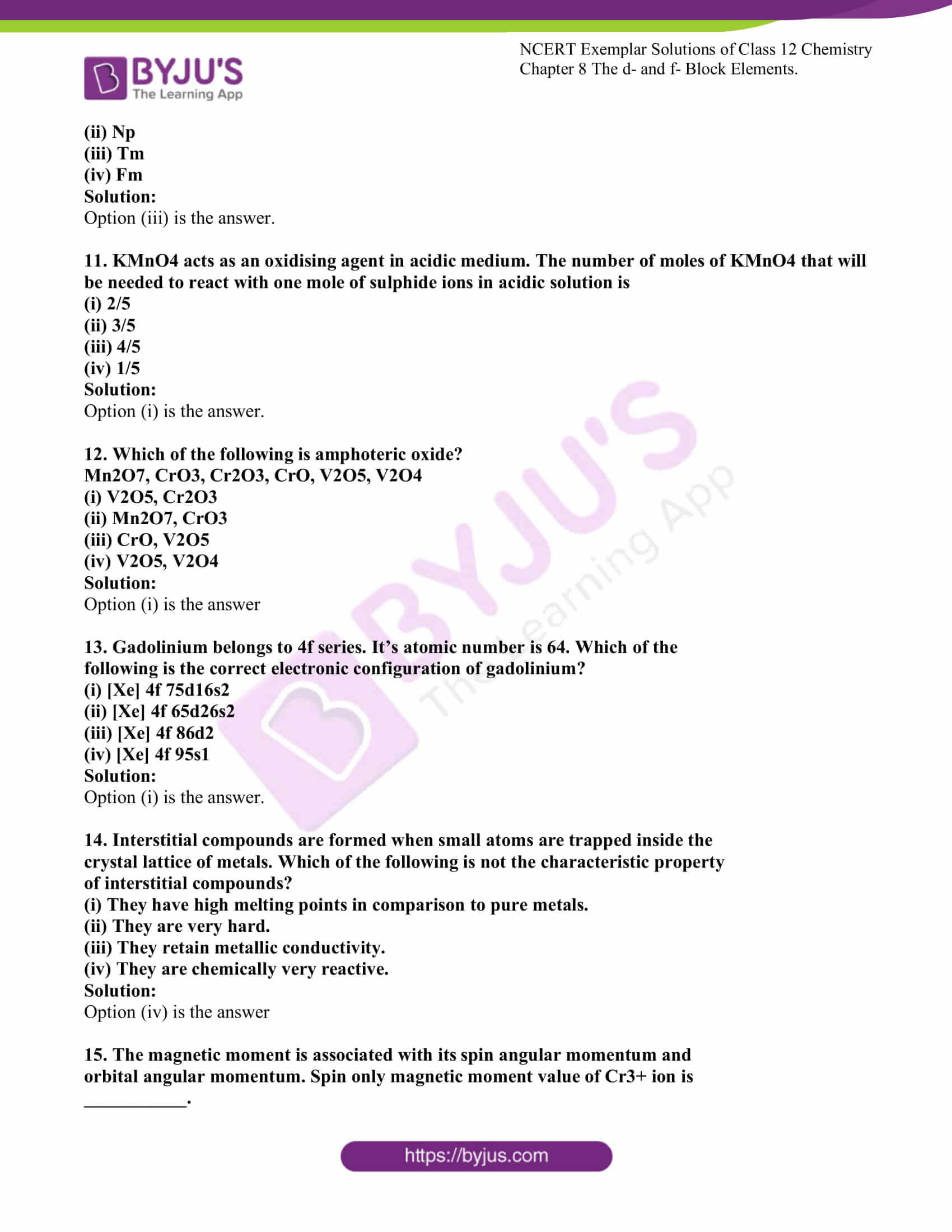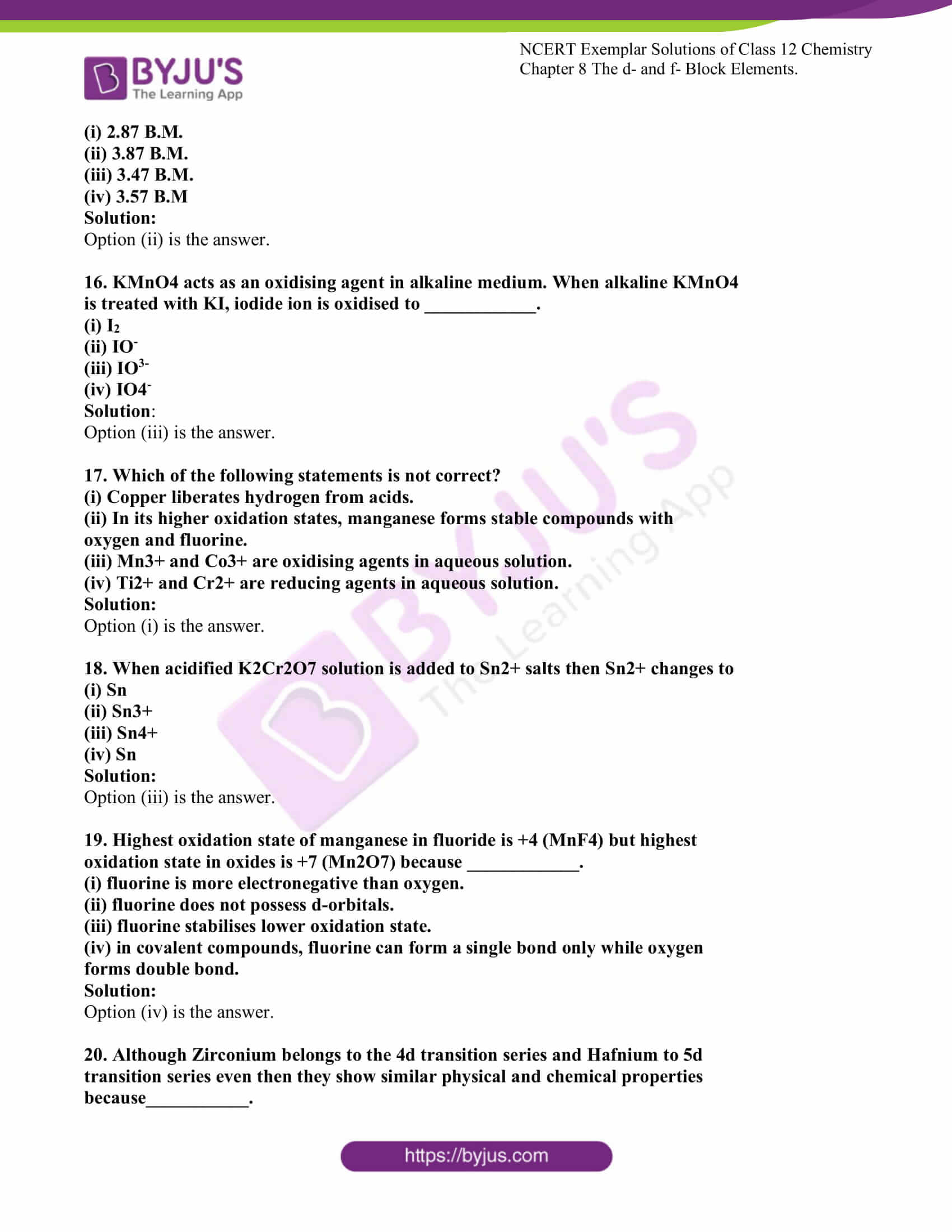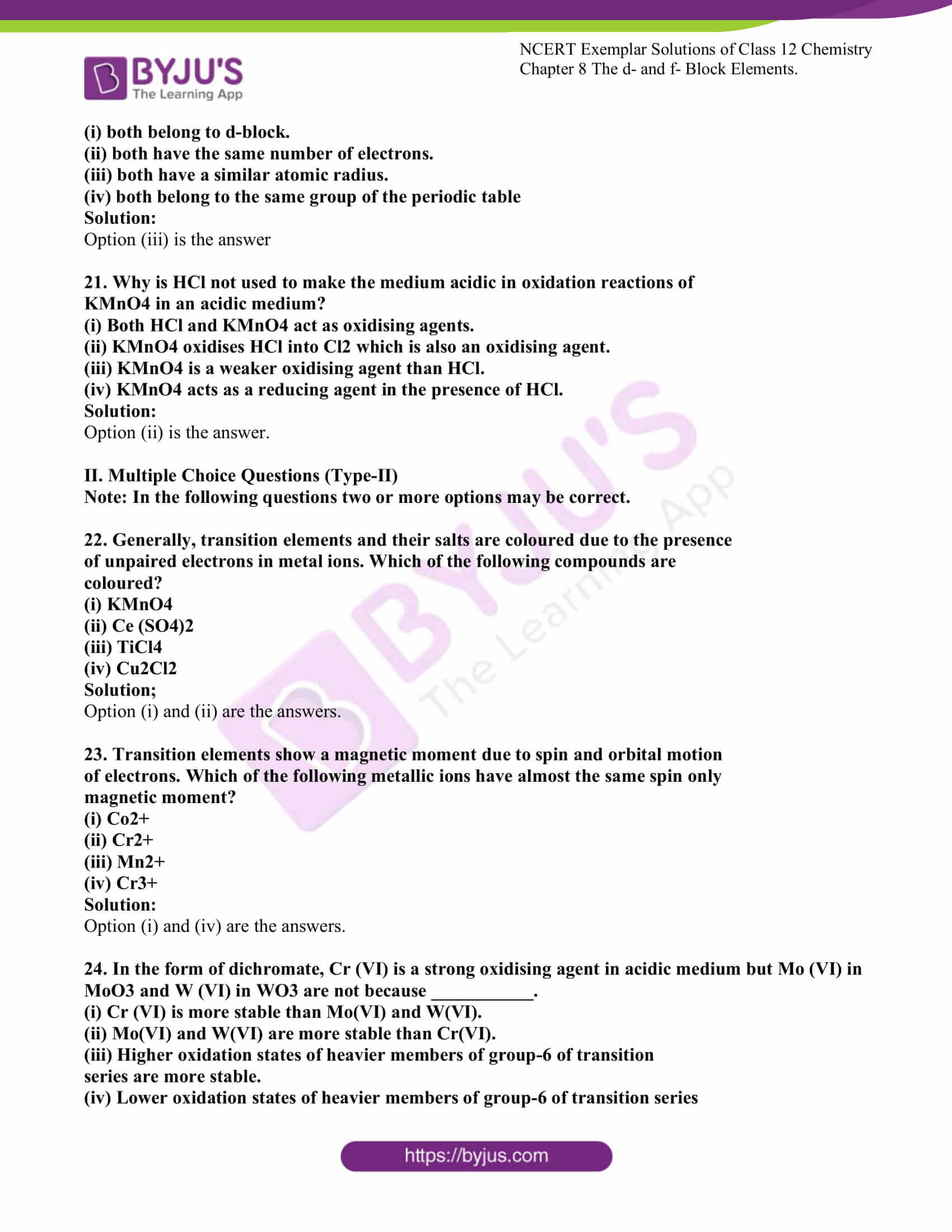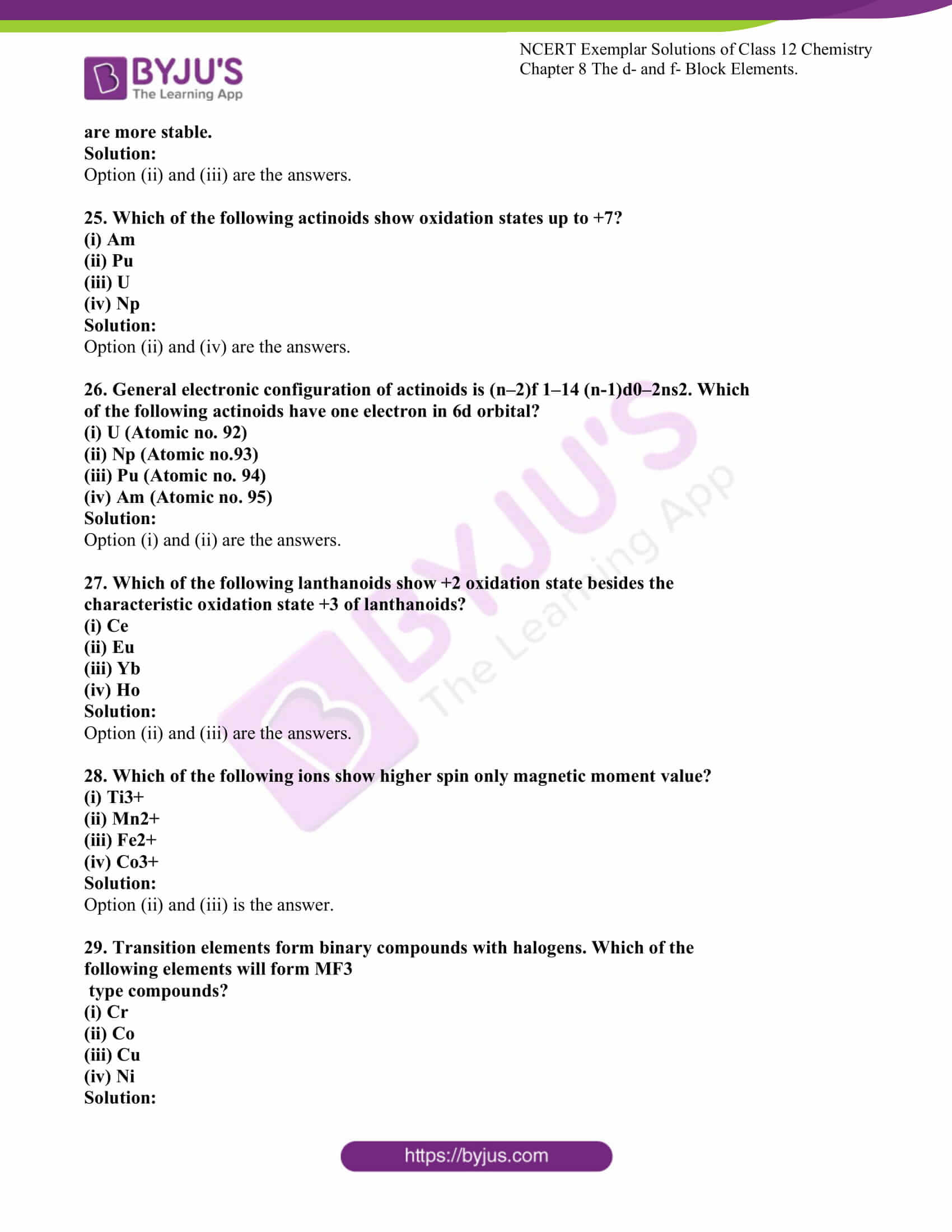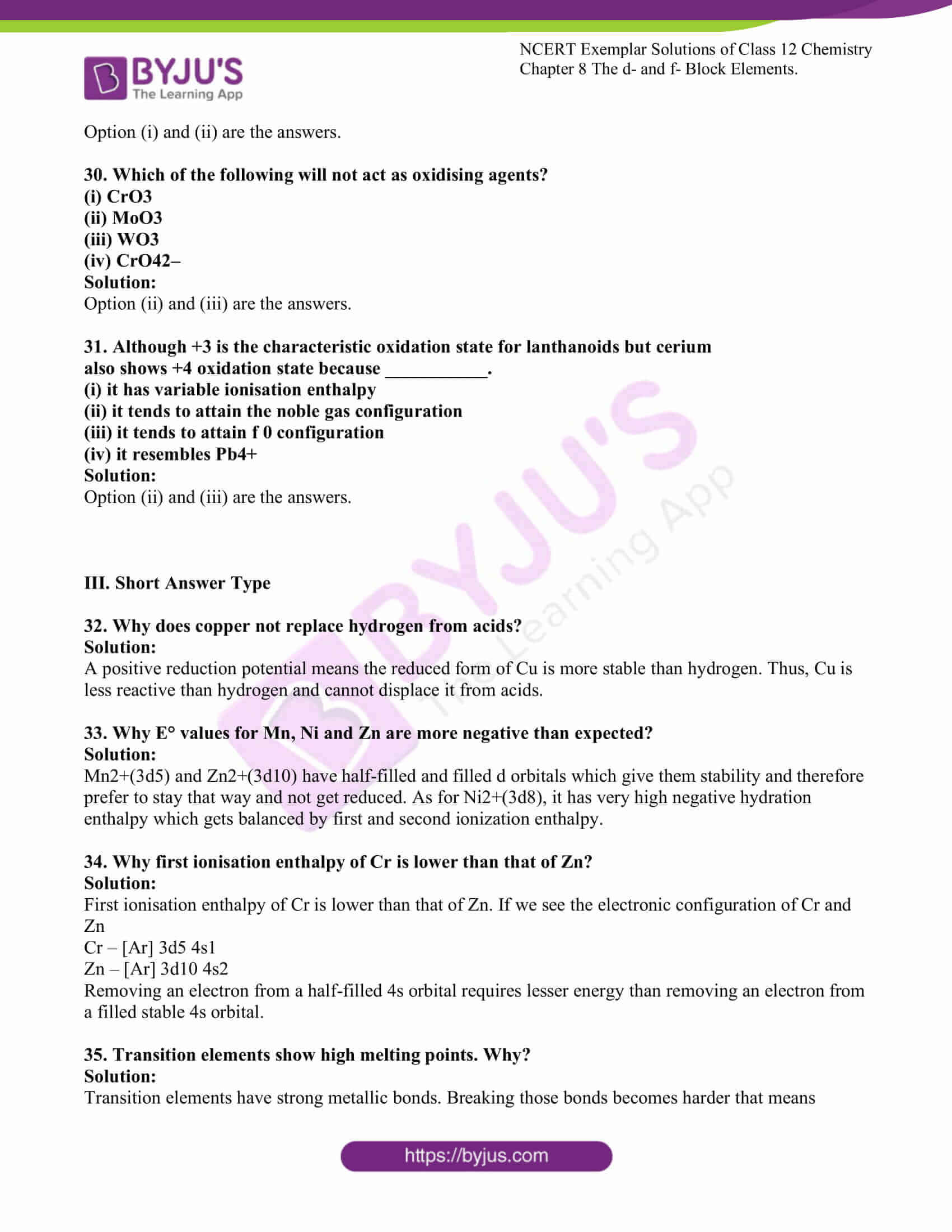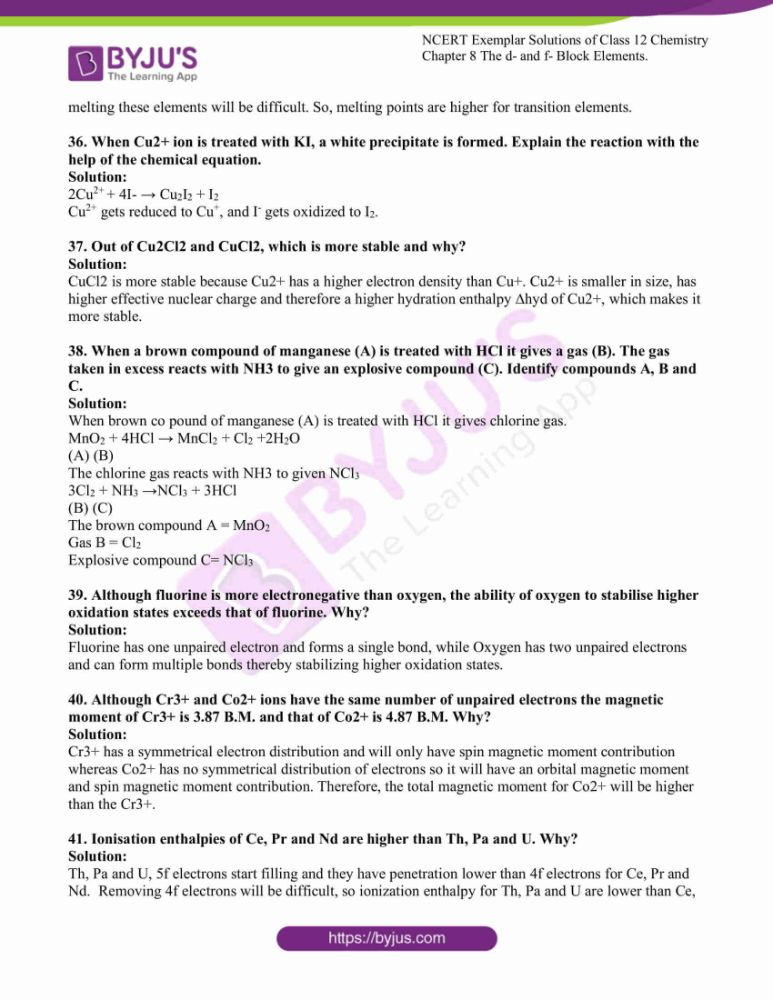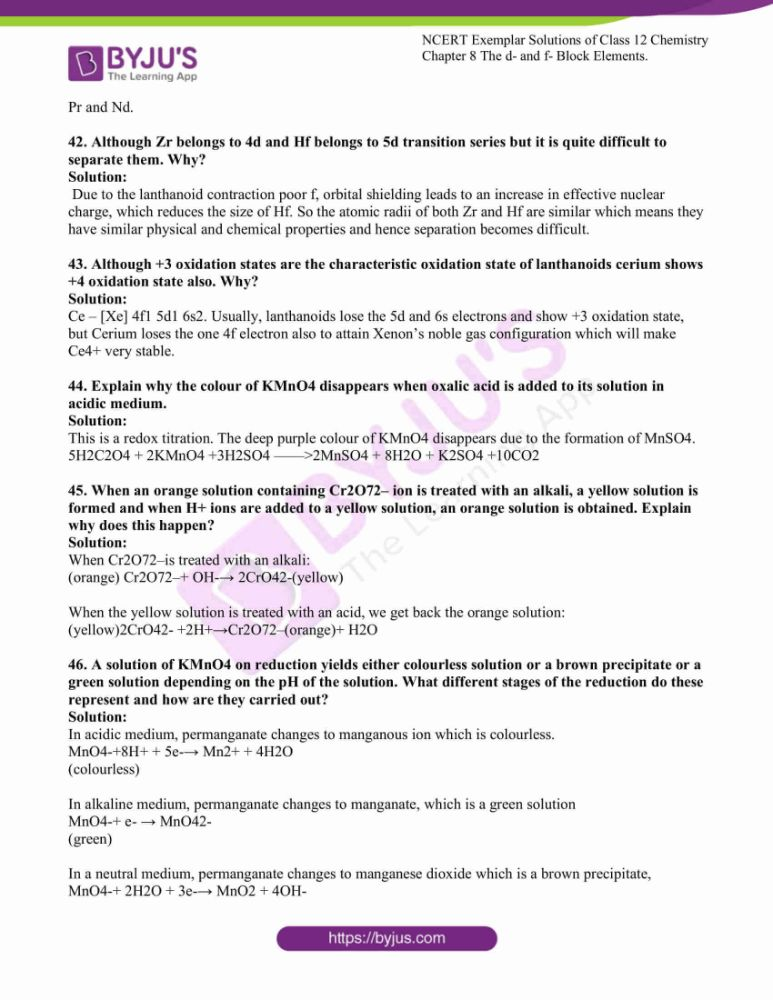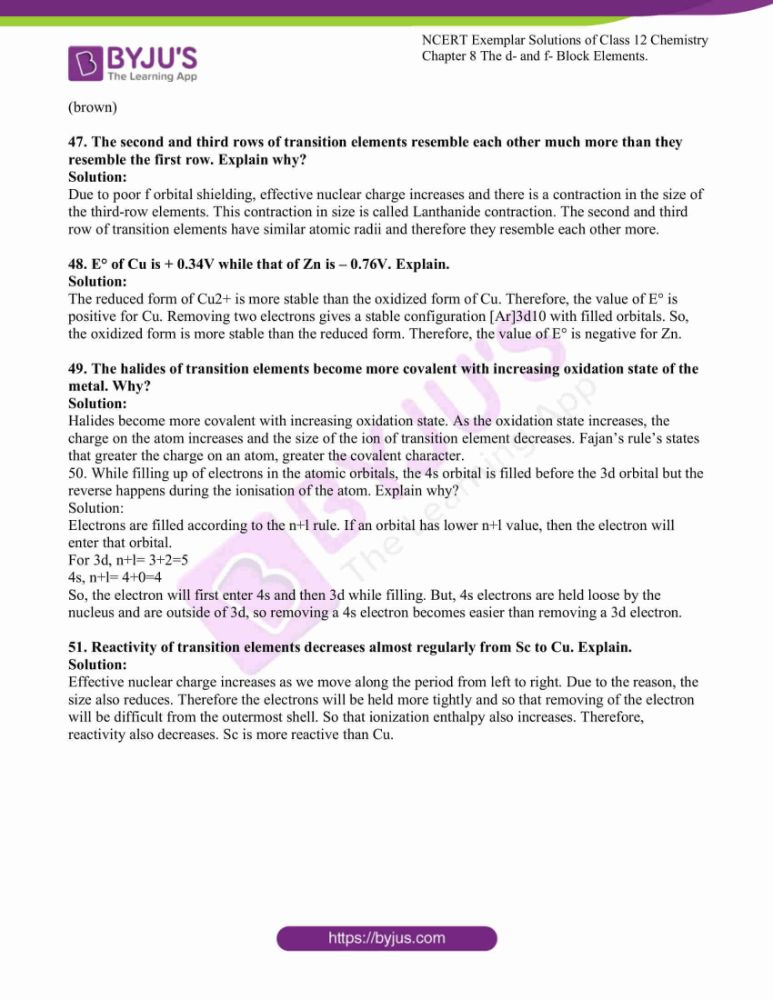### Access Solutions For NCERT Exemplar Class 12 Chemistry Chapter 8

I. Multiple Choice Questions (Type-I)

1. Electronic configuration of a transition element X in +3 oxidation state is

[Ar]3d5. What is its atomic number?

(i) 25

(ii) 26

(iii) 27

(iv) 24

Solution:

2. The electronic configuration of Cu(II) is 3d9 whereas that of Cu(I) is 3d10. Which

of the following is correct?

(i) Cu(II) is more stable

(ii) Cu(II) is less stable

(iii) Cu(I) and Cu(II) are equally stable

(iv) Stability of Cu(I) and Cu(II) depends on the nature of copper salts

Solution:

3. Metallic radii of some transition elements are given below. Which of these

elements will have the highest density?

 Element Metallic radii/pm Fe 126 Co 125 Ni 125 Cu 128

(i) Fe

(ii) Ni

(iii) Co

(iv) Cu

Solution:

4. Generally, transition elements form coloured salts due to the presence of unpaired electrons. Which of the following compounds will be coloured in solid-state?

(i) Ag2SO4

(ii) CuF2

(iii) ZnF2

(iv) Cu2Cl2

Solution:

5. On addition of a small amount of KMnO4 to concentrated H2SO4, a green oily compound is obtained which is highly explosive. Identify the compound from the following.

(i) Mn2O7

(ii) MnO2

(iii) MnSO4

(iv) Mn2O3

Solution;

6. The magnetic nature of elements depends on the presence of unpaired

electrons. Identify the configuration of transition element, which shows the highest

magnetic moment.

(i) 3d7

(ii) 3d5

(iii) 3d8

(iv) 3d2

Solution:

7. Which of the following oxidation state is common for all lanthanoids?

(i) +2

(ii) +3

(iii) +4

(iv) +5

Solution:

8. Which of the following reactions are disproportionation reactions?

(a) Cu+ → Cu2+ + Cu

(b) 3MnO4– + 4H+ → 2MnO4– + MnO2 + 2H2O

(c) 2KMnO4 → K2MnO4 + MnO2 + O2

(d) 2MnO4– + 3Mn2+ + 2H2O → 5MnO2 + 4H+(i) a, b

(ii) a, b, c

(iii) b, c, d

(iv) a, d

Solution:

9. When KMnO4 solution is added to the oxalic acid solution, the decolourisation is slow in the beginning but becomes instantaneous after some time because

(i) CO2 is formed as the product.

(ii) The reaction is exothermic.

(iii) MnO4– catalyses the reaction.

(iv) Mn2+ acts as an autocatalyst.

Solution:

10. There are 14 elements in the actinoid series. Which of the following elements does

not belong to this series?

(i) U

(ii) Np

(iii) Tm

(iv) Fm

Solution:

11. KMnO4 acts as an oxidising agent in acidic medium. The number of moles of KMnO4 that will be needed to react with one mole of sulphide ions in acidic solution is

(i) 2/5

(ii) 3/5

(iii) 4/5

(iv) 1/5

Solution:

12. Which of the following is amphoteric oxide?

Mn2O7, CrO3, Cr2O3, CrO, V2O5, V2O4

(i) V2O5, Cr2O3

(ii) Mn2O7, CrO3

(iii) CrO, V2O5

(iv) V2O5, V2O4

Solution:

13. Gadolinium belongs to 4f series. Its atomic number is 64. Which of the

following is the correct electronic configuration of gadolinium?

(i) [Xe] 4f 75d16s2

(ii) [Xe] 4f 65d26s2

(iii) [Xe] 4f 86d2

(iv) [Xe] 4f 95s1Solution:

14. Interstitial compounds are formed when small atoms are trapped inside the

crystal lattice of metals. Which of the following is not the characteristic property

of interstitial compounds?

(i) They have high melting points in comparison to pure metals.

(ii) They are very hard.

(iii) They retain metallic conductivity.

(iv) They are chemically very reactive.

Solution:

15. The magnetic moment is associated with its spin angular momentum and

orbital angular momentum. Spin only magnetic moment value of Cr3+ ion is

___________.

(i) 2.87 B.M.

(ii) 3.87 B.M.

(iii) 3.47 B.M.

(iv) 3.57 B.M

Solution:

16. KMnO4 acts as an oxidising agent in alkaline medium. When alkaline KMnO4

is treated with KI, iodide ion is oxidised to ____________.

(i) I2

(ii) IO

(iii) IO3-

(iv) IO4

Solution:

17. Which of the following statements is not correct?

(i) Copper liberates hydrogen from acids.

(ii) In its higher oxidation states, manganese forms stable compounds with

oxygen and fluorine.

(iii) Mn3+ and Co3+ are oxidising agents in aqueous solution.

(iv) Ti2+ and Cr2+ are reducing agents in aqueous solution.

Solution:

18. When acidified K2Cr2O7 solution is added to Sn2+ salts then Sn2+ changes to

(i) Sn

(ii) Sn3+

(iii) Sn4+

(iv) Sn

Solution:

19. Highest oxidation state of manganese in fluoride is +4 (MnF4) but highest

oxidation state in oxides is +7 (Mn2O7) because ____________.

(i) fluorine is more electronegative than oxygen.

(ii) fluorine does not possess d-orbitals.

(iii) fluorine stabilises lower oxidation state.

(iv) in covalent compounds, fluorine can form a single bond only while oxygen

forms double bond.

Solution:

20. Although Zirconium belongs to the 4d transition series and Hafnium to 5d

transition series even then they show similar physical and chemical properties

because___________.

(i) both belong to d-block.

(ii) both have the same number of electrons.

(iii) both have a similar atomic radius.

(iv) both belong to the same group of the periodic table

Solution:

21. Why is HCl not used to make the medium acidic in oxidation reactions of

KMnO4 in an acidic medium?

(i) Both HCl and KMnO4 act as oxidising agents.

(ii) KMnO4 oxidises HCl into Cl2 which is also an oxidising agent.

(iii) KMnO4 is a weaker oxidising agent than HCl.

(iv) KMnO4 acts as a reducing agent in the presence of HCl.

Solution:

II. Multiple Choice Questions (Type-II)

Note: In the following questions two or more options may be correct.

22. Generally, transition elements and their salts are coloured due to the presence

of unpaired electrons in metal ions. Which of the following compounds are

coloured?

(i) KMnO4

(ii) Ce (SO4)2

(iii) TiCl4

(iv) Cu2Cl2

Solution;

Option (i) and (ii) are the answers.

23. Transition elements show a magnetic moment due to spin and orbital motion

of electrons. Which of the following metallic ions have almost the same spin only

magnetic moment?

(i) Co2+

(ii) Cr2+

(iii) Mn2+

(iv) Cr3+

Solution:

Option (i) and (iv) are the answers.

24. In the form of dichromate, Cr (VI) is a strong oxidising agent in acidic medium but Mo (VI) in MoO3 and W (VI) in WO3 are not because ___________.

(i) Cr (VI) is more stable than Mo(VI) and W(VI).

(ii) Mo(VI) and W(VI) are more stable than Cr(VI).

(iii) Higher oxidation states of heavier members of group-6 of transition

series are more stable.

(iv) Lower oxidation states of heavier members of group-6 of transition series

are more stable.

Solution:

Option (ii) and (iii) are the answers.

25. Which of the following actinoids show oxidation states up to +7?

(i) Am

(ii) Pu

(iii) U

(iv) Np

Solution:

Option (ii) and (iv) are the answers.

26. General electronic configuration of actinoids is (n–2)f 1–14 (n-1)d0–2ns2. Which

of the following actinoids have one electron in 6d orbital?

(i) U (Atomic no. 92)

(ii) Np (Atomic no.93)

(iii) Pu (Atomic no. 94)

(iv) Am (Atomic no. 95)

Solution:

Option (i) and (ii) are the answers.

27. Which of the following lanthanoids show +2 oxidation state besides the

characteristic oxidation state +3 of lanthanoids?

(i) Ce

(ii) Eu

(iii) Yb

(iv) Ho

Solution:

Option (ii) and (iii) are the answers.

28. Which of the following ions show higher spin only magnetic moment value?

(i) Ti3+

(ii) Mn2+

(iii) Fe2+

(iv) Co3+

Solution:

Option (ii) and (iii) is the answer.

29. Transition elements form binary compounds with halogens. Which of the

following elements will form MF3

type compounds?

(i) Cr

(ii) Co

(iii) Cu

(iv) Ni

Solution:

Option (i) and (ii) are the answers.

30. Which of the following will not act as oxidising agents?

(i) CrO3

(ii) MoO3

(iii) WO3

(iv) CrO42–Solution:

Option (ii) and (iii) are the answers.

31. Although +3 is the characteristic oxidation state for lanthanoids but cerium

also shows +4 oxidation state because ___________.

(i) it has variable ionisation enthalpy

(ii) it tends to attain the noble gas configuration

(iii) it tends to attain f 0 configuration

(iv) it resembles Pb4+

Solution:

Option (ii) and (iii) are the answers.

32. Why does copper not replace hydrogen from acids?

Solution:

A positive reduction potential means the reduced form of Cu is more stable than hydrogen. Thus, Cu is less reactive than hydrogen and cannot displace it from acids.

33. Why E° values for Mn, Ni and Zn are more negative than expected?

Solution:

Mn2+(3d5) and Zn2+(3d10) have half-filled and filled d orbitals which give them stability and therefore prefer to stay that way and not get reduced. As for Ni2+(3d8), it has very high negative hydration enthalpy which gets balanced by first and second ionization enthalpy.

34. Why first ionisation enthalpy of Cr is lower than that of Zn?

Solution:

First ionisation enthalpy of Cr is lower than that of Zn. If we see the electronic configuration of Cr and Zn

Cr – [Ar] 3d5 4s1

Zn – [Ar] 3d10 4s2

Removing an electron from a half-filled 4s orbital requires lesser energy than removing an electron from a filled stable 4s orbital.

35. Transition elements show high melting points. Why?

Solution:

Transition elements have strong metallic bonds. Breaking those bonds becomes harder that means melting these elements will be difficult. So, melting points are higher for transition elements.

36. When Cu2+ ion is treated with KI, a white precipitate is formed. Explain the reaction with the help of the chemical equation.

Solution:

2Cu2+ + 4I- → Cu2I2 + I2

Cu2+ gets reduced to Cu+, and I gets oxidized to I2.

37. Out of Cu2Cl2 and CuCl2, which is more stable and why?

Solution:

CuCl2 is more stable because Cu2+ has a higher electron density than Cu+. Cu2+ is smaller in size, has higher effective nuclear charge and therefore a higher hydration enthalpy ∆hyd of Cu2+, which makes it more stable.

38. When a brown compound of manganese (A) is treated with HCl it gives a gas (B). The gas taken in excess reacts with NH3 to give an explosive compound (C). Identify compounds A, B and C.

Solution:

When brown co pound of manganese (A) is treated with HCl it gives chlorine gas.

MnO2 + 4HCl → MnCl2 + Cl2 +2H2O

(A) (B)

The chlorine gas reacts with NH3 to given NCl3

3Cl2 + NH3 →NCl3 + 3HCl

(B) (C)

The brown compound A = MnO2

Gas B = Cl2

Explosive compound C= NCl3

39. Although fluorine is more electronegative than oxygen, the ability of oxygen to stabilise higher oxidation states exceeds that of fluorine. Why?

Solution:

Fluorine has one unpaired electron and forms a single bond, while Oxygen has two unpaired electrons and can form multiple bonds thereby stabilizing higher oxidation states.

40. Although Cr3+ and Co2+ ions have the same number of unpaired electrons the magnetic moment of Cr3+ is 3.87 B.M. and that of Co2+ is 4.87 B.M. Why?

Solution:

Cr3+ has a symmetrical electron distribution and will only have spin magnetic moment contribution whereas Co2+ has no symmetrical distribution of electrons so it will have an orbital magnetic moment and spin magnetic moment contribution. Therefore, the total magnetic moment for Co2+ will be higher than the Cr3+.

41. Ionisation enthalpies of Ce, Pr and Nd are higher than Th, Pa and U. Why?

Solution:

Th, Pa and U, 5f electrons start filling and they have penetration lower than 4f electrons for Ce, Pr and Nd. Removing 4f electrons will be difficult, so ionization enthalpy for Th, Pa and U are lower than Ce, Pr and Nd.

42. Although Zr belongs to 4d and Hf belongs to 5d transition series but it is quite difficult to separate them. Why?

Solution:

Due to the lanthanoid contraction poor f, orbital shielding leads to an increase in effective nuclear charge, which reduces the size of Hf. So the atomic radii of both Zr and Hf are similar which means they have similar physical and chemical properties and hence separation becomes difficult.

43. Although +3 oxidation states are the characteristic oxidation state of lanthanoids cerium shows +4 oxidation state also. Why?

Solution:

Ce – [Xe] 4f1 5d1 6s2. Usually, lanthanoids lose the 5d and 6s electrons and show +3 oxidation state, but Cerium loses the one 4f electron also to attain Xenon’s noble gas configuration which will make Ce4+ very stable.

44. Explain why the colour of KMnO4 disappears when oxalic acid is added to its solution in acidic medium.

Solution:

This is a redox titration. The deep purple colour of KMnO4 disappears due to the formation of MnSO4.

5H2C2O4 + 2KMnO4 +3H2SO4 ——>2MnSO4 + 8H2O + K2SO4 +10CO2

45. When an orange solution containing Cr2O72– ion is treated with an alkali, a yellow solution is formed and when H+ ions are added to a yellow solution, an orange solution is obtained. Explain why does this happen?

Solution:

When Cr2O72–is treated with an alkali:

(orange) Cr2O72–+ OH-→ 2CrO42-(yellow)

When the yellow solution is treated with an acid, we get back the orange solution:

(yellow)2CrO42- +2H+→Cr2O72–(orange)+ H2O

46. A solution of KMnO4 on reduction yields either colourless solution or a brown precipitate or a green solution depending on the pH of the solution. What different stages of the reduction do these represent and how are they carried out?

Solution:

In acidic medium, permanganate changes to manganous ion which is colourless.

MnO4-+8H+ + 5e-→ Mn2+ + 4H2O

(colourless)

In alkaline medium, permanganate changes to manganate, which is a green solution

MnO4-+ e- → MnO42-

(green)

In a neutral medium, permanganate changes to manganese dioxide which is a brown precipitate,

MnO4-+ 2H2O + 3e-→ MnO2 + 4OH-

(brown)

47. The second and third rows of transition elements resemble each other much more than they resemble the first row. Explain why?

Solution:

Due to poor f orbital shielding, effective nuclear charge increases and there is a contraction in the size of the third-row elements. This contraction in size is called Lanthanide contraction. The second and third row of transition elements have similar atomic radii and therefore they resemble each other more.

48. E° of Cu is + 0.34V while that of Zn is – 0.76V. Explain.

Solution:

The reduced form of Cu2+ is more stable than the oxidized form of Cu. Therefore, the value of E° is positive for Cu. Removing two electrons gives a stable configuration [Ar]3d10 with filled orbitals. So, the oxidized form is more stable than the reduced form. Therefore, the value of E° is negative for Zn.

49. The halides of transition elements become more covalent with increasing oxidation state of the metal. Why?

Solution:

Halides become more covalent with increasing oxidation state. As the oxidation state increases, the charge on the atom increases and the size of the ion of transition element decreases. Fajan’s rule’s states that greater the charge on an atom, greater the covalent character.

50. While filling up of electrons in the atomic orbitals, the 4s orbital is filled before the 3d orbital but the reverse happens during the ionisation of the atom. Explain why?

Solution:

Electrons are filled according to the n+l rule. If an orbital has lower n+l value, then the electron will enter that orbital.

For 3d, n+l= 3+2=5

4s, n+l= 4+0=4

So, the electron will first enter 4s and then 3d while filling. But, 4s electrons are held loose by the nucleus and are outside of 3d, so removing a 4s electron becomes easier than removing a 3d electron.

51. Reactivity of transition elements decreases almost regularly from Sc to Cu. Explain.

Solution:

Effective nuclear charge increases as we move along the period from left to right. Due to the reason, the size also reduces. Therefore the electrons will be held more tightly and so that removing of the electron will be difficult from the outermost shell. So that ionization enthalpy also increases. Therefore, reactivity also decreases. Sc is more reactive than Cu.

Important concepts of Chapter 8 D and F Block elements

1. Position in the Periodic Table –
2. Electronic Configurations of the d-Block Elements
3. General Properties of the Transition Elements (d-Block)
4. Some Important Compounds of Transition Elements
5. The Lanthanides
6. The Actinides
7. Some Applications of d- and f-Block Elements.

To download NCERT Class 12 Chemistry Exemplar for Chapter 8 D and F Block elements visit the below link

### Why Opt for BYJU’S?

BYJU’S is India’s top online education providers which use highly efficient interactive way of learning. BYJU’S provides notes, study materials, NCERT Solutions, previous year question papers, sample papers, videos and animations that help you not only in understanding the topic but also help you in remembering the concepts for a very long time.